Problem: Find the probability that the length of a random chord will be greater than the side of an equilateral triangle inscribed in the circle.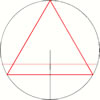First solution:
A chord is fully determined by its midpoint. The chords whose length exceeds the side of an equilateral triangle have their midpoints closer to the center than half the radius, the probability becomes 1/2.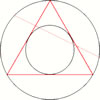Second solution:
A chord is fully determined by its midpoint. The chords whose length exceeds the side of an equilateral triangle have their midpoints inside a smaller circle with the radius equal to 1/2 of the given one. Hence, its area is 1/4 of the big circle which also defines the proportion of favorable outcomes - 1/4.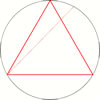Third solution:
A chord is fully determined by its endpoint. If we use one vertex of the triangle as the first point, the chords whose length exceeds the side of an equilateral triangle have their endpoints on the arc between two opposite vertices, the probability becomes 1/3.
Is it a paradox or just a blunder? Does it have a unique solution? Is it a well-posed problem? Is it allowed to translate chords onto the surface and why do we do that? How do we choose the surface shape in that case?
The problem was proposed by French mathematician Joseph Bertrand in 1889. The offical statement is that it is a paradox and at the same time a good example that words "chosen randomly" sometimes are not enough. In other words, we must know how to find probability. The explanation for statement is that there are a lot of different results of the problem which depend on the method of calculation. Therefore, this paradox has became the model for explanation when we can't determine something, specially in theory of probability. Explanation on this page is realized like a dialogue between one fictive visitor and me (V-visitor, B-that is me, Božur), and we are discussing about this paradox from first example to the third. You'll see, there is no need for complex math calculations.
Yet, when you are confused about those examples, we can go to read dialogue about the first. Chords that are longer then side of equilateral triangle are red. Explanation is realized like a dialogue between one fictive visitor and me.
Explanation of the first example:
A chord is fully determined by its midpoint. The chords whose length exceeds the side of an equilateral triangle have their midpoints closer to the center than half the radius, the probability becomes 1/2.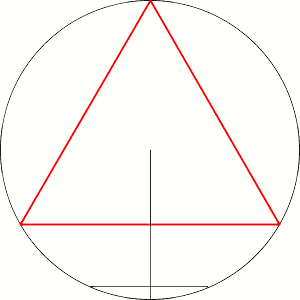DIALOGUE:
V: Ok, I saw one simulation in java that shows the same result like this!
B: Could program make anything different what we order them to do?
V: I don't understand.
B: Well, program execute some math equation, if we write 2x3 program will multiple it.
V: And, if we use wrong calculation we will get wrong result.
B: Exactly!
V: Then, why we are using them.
B: You mean computers?
V: Yes!
B: Only for faster calculation.
V: And what is wrong here?
B: Let's find out that together. What is idea here?
V: One point of the surface of the circle determine middle point of one chord. And if the point stays closer to the center than half the radius then is appropriate chord longer then side of inscribed equilateral triangle.
B: What do you mean, why is radius used here?
V: Because, it includes set of chords from shortest one to longest.
B: Is that only reason?
V: Yes! If it represents set of chords that we have here, that is enough.
B: We can use then and some other line instead radius. Like on the pictures.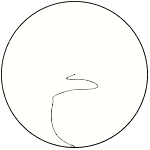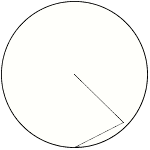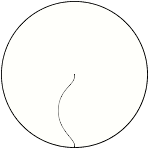V: But it gives different result.
B: And?
V: It can't covers surface of the circle uniformly. With radius we can calculate on that way because the circle consist of infinity radii.
B: Well, if we can't cover surface uniformly, we can't take that line in calculation?
V: Yes.
B: How we calculate surface of one circle or one part of the circle? How we get the formula for that?
V: With integration.
B: And what we integrate there? Radii?
V: If we integrate radii we will not get formula for surface of the circle!
B: Thus, what?
V: I see! We integrate little sectors.
B: And that isn't the same.
V: No, it is not! But if we leave the angle very small we will get the radius.
B: Only if angle has zero value we will get the radius!
V: Then it is not a sector any more! 100x0 equals 0, and we can't make sector.
B: So, could we cover with infinity radii circle shape.
V: No, we can't! Then is all wrong, and it is the same as we use that curves above for calculation.
B: Correct!
V: So, we must calculate with sectors, to make in summary a circle?
B: That is problem of second example, let's go there.
Explanation of the second example:
A chord is fully determined by its midpoint. The chords whose length exceeds the side of an equilateral triangle have their midpoints inside a smaller circle with radius equal to 1/2 that of the given one. Hence, its area is 1/4 of the big circle which also defines the proportion of favourable outcomes - 1/4.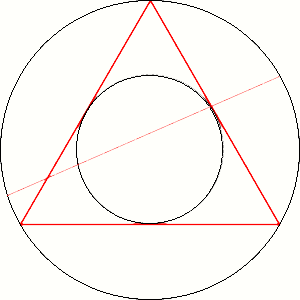DIALOGUE:
V: Like previous example we must analyse correctness of this statement.
B: Of course!
V: I don't know what to ask, it looks ok. Here is the surface covered uniformly.
B: Try something!
V: Hm, why the surface here must be flat? Why we don't copy midpoint to uneven surface, and then look for their rates?
B: It is very good question. Why not some irregular shape in space like on those pictures below, where is the difference?V: Maybe, because it is minimum value of surface?
B: And why is that so important?
V: It seems in that way when we seek where falls midpoint on some irregular surface we will make some of the regions more probably then other. We can give for inner region better chance if is there larger surface like on picture at right side.
B: Why we can't do that?
V: Because we can't change their rates!
B: Haven't we that in statement of our example 2?
V: No!
B: How that?
V: All of the chords are determined by only one point of the surface of circle.
B: And the middle point?
V: It has also their chord!
B: Only one?
V: Oh, yes! There is unlimited number of chords that is determined by middle point!
B: And what do you think now?
V: Yes, but that is only one point, we can neglect it! Probability of choosing one point is zero in this case when we have infinity points!
B: Points or chords?
V: Sorry?
B: Problem is to calculate probability of choosing chords not points!
V: And?
B: Could we neglect unlimited number of chords?
V: Definitely not! If we do that we can also use in our calculations those irregular shapes above.
B: That is my opinion too.
V: So, here we have wrong model because we do not know what is relation between our one chord and one point here.
B: Yes that is wrong model but likable.
V: I haven't more question for this example.
B: Maybe some of the visitors will have and make dialogue for this part longer. Let's go to the third example.

Explanation of the third example:
A chord is fully determined by its endpoint. If we use one vertex of the triangle as the first point, the chords whose length exceeds the side of an equilateral triangle have their endpoints on the arc between two opposite vertices, the probability becomes 1/3.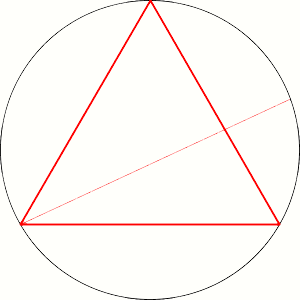DIALOGUE:
V: Here we calculate probability by using one point, and then we are looking where could be the second. Is that correct if we are looking for just one point?
B: If we get same results for any point, it does not matter. Probability are not get changed if any point gives same result.
V: Ok.
B: Now, try to find where is mistake.
V: Hm, it is difficult.
B: Why?
V: In previous samples we had hidden irregular transformation.
B: Maybe here is hidden too.
V: Maybe. But here it looks so simple.
B: Be little precisely.
V: Well, in first example the middle point of chord is used to transform our set of chords to one radius. In second it is used to transform our set to the surface of the circle. And here we do not use anything.
B: But we are using endpoint of the chord.
V: Yes, but not for some transformation, we just counting chords here!
B: Haven't we here transformation?
V: No, we haven't!
B: But, here we replace one endpoint with one chord. That is transformation too!
V: You are a hair-splitter.
B: That is important.
V: Here we replace one chord with one point.
B: It is called bijection! And what we have in previous examples?
V: In the first example we also have bijection to one radius but there is one more transformation.
B: Which ones?
V: When we say that all midpoints are on all radii, but all midpoints can only be on all little sectors! If they are not, we are neglecting some of them in our calculations.
B: Bravo! And the second example?
V: We have discussed yet about that. There is problem the center of the circle, it involves transformation that is not bijection. We are neglecting there infinity number of chords.
B: Exactly.
V: It seems then statement for this example is correct. And if someone find in the future some more different examples?
B: There can be more examples but if we don't make some irregulars transformation in calculation, the result must be the same.
V: On many web pages about Bertrand's paradox I have found statements that the problem in Bertrand's paradox is to define on which way should we try to find probability. And because it is not clearly defined we have paradox here.
B: The problem is very clearly defined, and we have found mistakes in previous examples. Look at pyramid below. Suppose, we have to find probability that the randomly chosen point belongs to red area of pyramid. Could we say it depends of our point of view, and take all of that results as correct? Is that paradox too?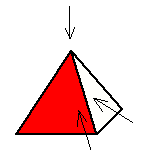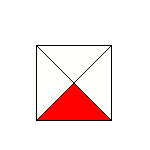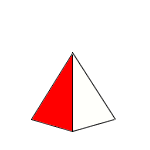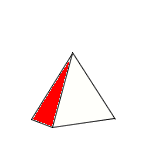V: Well, that is the answer of Bertrand's paradox. It is a blunder!
B: That is correct. It is not a paradox and the result is 1/3!
Conclusion:
That is all.
So called Bertrand's paradox is not a paradox because there is only one correct solution for the problem and it is 1/3!
If you don't agree, you can add your comment to the discussion bellow. Here is the deal: If you don't enter a comment that will be accepted like agreement :). Of course, positive comments are also welcome.

You can contact me directly via bozur.vujicic(et)gmail.com, but if you want to discuss this Bertrand's paradox, please use the form below. Maybe your thoughts can be interesting to others!